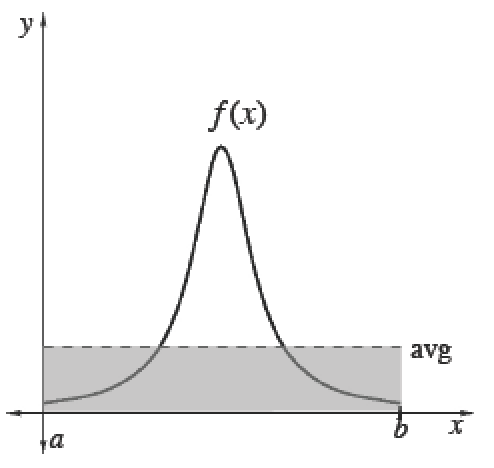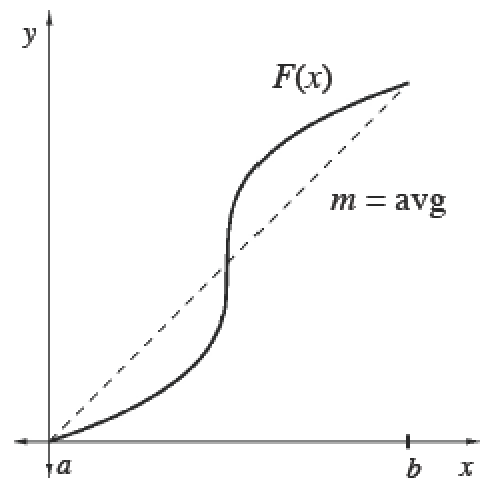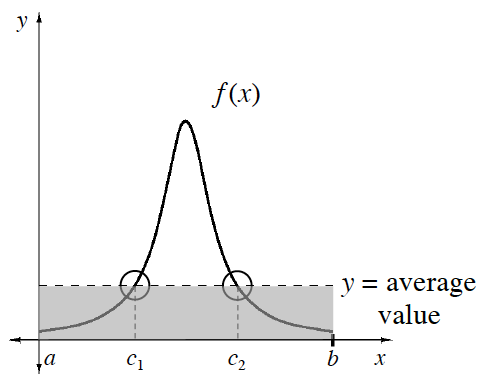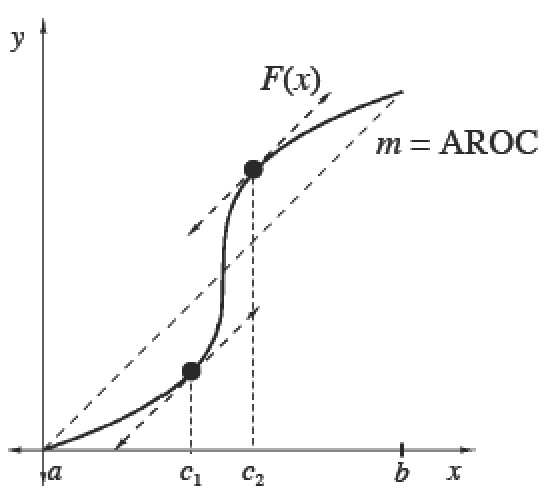### Home > CALC3RD > Chapter Ch7 > Lesson 7.1.1 > Problem7-8

7-8.

For each function below, calculate the average value over the given interval and state the value of $t$ such that $g\left(t\right)$ equals the average value.

1. $g(t) = 3t + 6 \text{ for } [0, 8]$

Read the Math Note about how to compute the Mean Value of $g\left(x\right)$, given $g\left(x\right)$.

To find the the time the function is at its average value, let $g\left(t\right) =$ the average value and solve for $t$.

1. $g(t) = 3e^t \text{ for } [0, 1]$

Average Value $= 3e − 3$. Now find the time, $t$, that $g\left(t\right) =$ its average value.

##### Average (Mean) Values
 To calculate the mean (average) value of a finite set of items, add up the values of items and divide by the number of items.Integrals help us add over a continuous interval. Therefore, for any continuous function $f$ :$\frac{\int_a^bf(x)dx}{b-a}=$ mean value of $f$ over $[a, b]$Since $\int _ { a } ^ { b } f ( x ) d x$, we can also calculate the average value of any function $f$ using its antiderivative $F$. Its average slope gives the average rate of change of $F$, which is the same as the average value of $f.$$\frac{\int_a^bf(x)dx}{b-a}=\frac { F ( b ) - F ( a ) } { b - a }=$  mean rate of change of $F ′$ over $[a, b]$The Mean Value Theorem states that a differentiable function will reach its average (mean) value at least once on any closed interval.
Check your values of t in parts (a) and (b). Are they within the the given closed intervals?

The Mean Value Theorem

 The Mean Value Theorem for IntegralsIf $f$ is continuous on $[a, b]$, then there exists at least one point $x = c$ in $\left(a, b\right)$ such that $\frac { 1 } { b - a } \int _ { a } ^ { b } f ( x ) d x= f(c)$.The Mean Value Theorem for DerivativesIf $F$ is continuous on $[a, b]$ and differentiable on $\left(a, b\right)$, then there exists at least one point $x = c$ in $\left(a, b\right)$ such that $F ^ { \prime } ( c ) = \frac { F ( b ) - F ( a ) } { b - a } = f ( c )$.